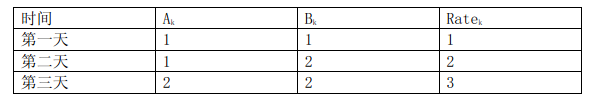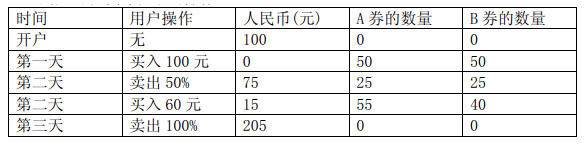# #NOI2007B. 货币兑换

ID: 111 Type: Default 1000ms 256MiB Tried: 4 Accepted: 4 Difficulty: 10 Uploaded By: Tags>NOI2007

# 货币兑换

## 题目描述

$Y$ 最近在一家金券交易所工作。该金券交易所只发行交易两种金券： $A$ 纪念券（以下简称 $A$ 券）和 $B$ 纪念券（以下简称 $B$ 券）。每个持有金券的顾客都有一个自己的帐户。金券的数目可以是一个实数。每天随着市场的起伏波动，两种金券都有自己当时的价值，即每一单位金券当天可以兑换的人民币数目。我们记录第 $K$ 天中 $A$ 券和 $B$ 券的价值分别为 $A_K$$B_K$（元/单位金券）。为了方便顾客，金券交易所提供了一种非常方便的交易方式：比例交易法。比例交易法分为两个方面：

• 卖出金券：顾客提供一个 $[0,100]$ 内的实数 $\text{OP}$ 作为卖出比例，其意义为：将 $\text{OP}\%$$A$ 券和 $\text{OP}\%$$B$ 券以当时的价值兑换为人民币；
• 买入金券：顾客支付 $\text{IP}$ 元人民币，交易所将会兑换给用户总价值为 $\text{IP}$ 的金券，并且，满足提供给顾客的 $A$ 券和 $B$ 券的比例在第 $K$ 天恰好为 $\text{Rate}_K$ ；例如，假定接下来 $3$ 天内的 $A_k , B_k , \text{Rate}_K$ 的变化分别为：## 输出格式

3 100
1 1 1
1 2 2
2 2 3

225.000


## 数据范围与提示

#### 提示

• 输入文件可能很大，请采用快速的读入方式。
• 必然存在一种最优的买卖方案满足：每次买进操作使用完所有的人民币；每次卖出操作卖出所有的金券。